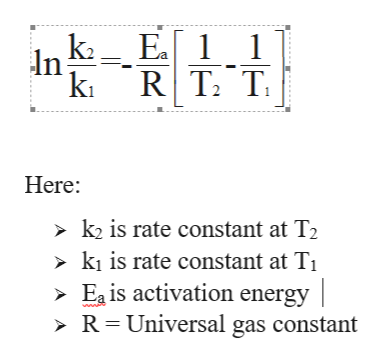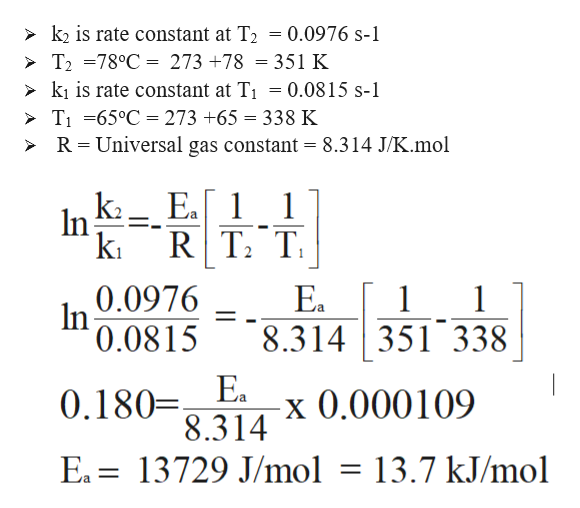# A first-order reaction has a rate constant k = 0.0815 s-1 at a temperature of 65oC and a rate constant k = 0.0976 s-1 at a temperature of 78oC. What is the activation energy of this reaction in kJ/mole?

Question
79 views

A first-order reaction has a rate constant k = 0.0815 s-1 at a temperature of 65oC and a rate constant k = 0.0976 s-1 at a temperature of 78oC. What is the activation energy of this reaction in kJ/mole?

check_circle

Step 1

To calculate activation energy of the reaction in kJ/mole with the given rate constant at different temperature values, the formula for first order kinetic is:help_outlineImage Transcriptioncloseka_ Ea 1 In ki R T2 T Here k2 is rate constant at T2 > ki is rate constant at T Ea is activation energy | > R = Universal gas constant A A A fullscreen
Step 2

Plug the given values to cal...help_outlineImage Transcriptionclose0.0976 s- > k2 is rate constant at T2 > T2 =78°C = 273 +78 = 351 K > ki is rate constant at T > TI 65°C = 273 +65 = 338 K > R Universal gas constant = 8.314 J/K.mol 0.0815 s- Ea 1 1 k R T2 T 1 1 0.0976 In 0.0815 Ea 1 8.314351 338 0.180 8.314 E x0.000109 )= EA 13729 J/mol = 13.7 kJ/mol fullscreen

### Want to see the full answer?

See Solution

#### Want to see this answer and more?

Solutions are written by subject experts who are available 24/7. Questions are typically answered within 1 hour.*

See Solution
*Response times may vary by subject and question.
Tagged in

### Chemical Kinetics